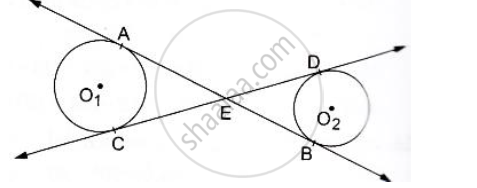# In the Given Figure Common Tangents Ab and Cd to the Two Circles with Centres O1 and O2 Intersect at E. Prove that Ab=Cd - Mathematics

In the given figure common tangents AB and CD to the two circles with centres O1 and O2 intersect at E. Prove that AB=CD#### Solution

We know that tangent segments to a circle from the same external point are congruent.
So, we have
EA = EC for the circle having center O1
and
ED = EB for the circle having center O
Now, Adding ED on both sides in EA = EC. we get
EA+ ED  =  EC + ED
⇒  EA + EB = EC + ED
⇒  AB = CD

Concept: Concept of Circle - Centre, Radius, Diameter, Arc, Sector, Chord, Segment, Semicircle, Circumference, Interior and Exterior, Concentric Circles
Is there an error in this question or solution?